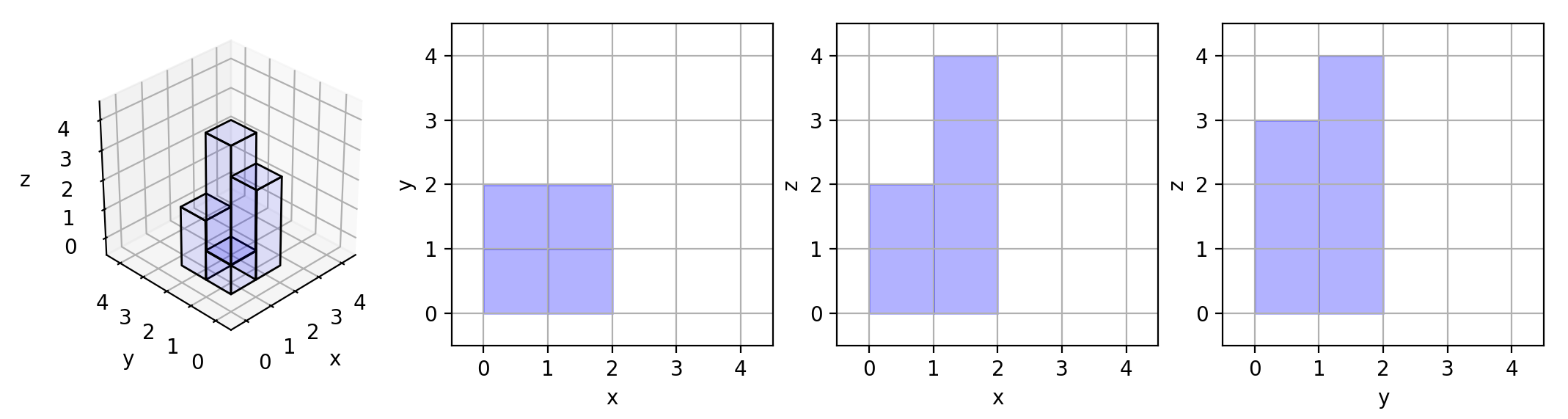# 883. Projection Area of 3D Shapes 三维形体投影面积

@TOC

## # 题目描述

On a `N * N` grid, we place some `1 * 1 * 1` cubes that are axis-aligned with the x, y, and z axes.

Each value `v = grid[i][j]` represents a tower of v cubes placed on top of grid cell `(i, j)`.

Now we view the projection of these cubes onto the xy, yz, and zx planes.

A projection is like a shadow, that maps our 3 dimensional figure to a 2 dimensional plane.

Here, we are viewing the "shadow" when looking at the cubes from the top, the front, and the side.

Return the total area of all three projections.

Example 1:

``````Input: []
Output: 5
``````

Example 2:

``````Input: [[1,2],[3,4]]
Output: 17
Explanation:
Here are the three projections ("shadows") of the shape made with each axis-aligned plane.
``````Example 3:

``````Input: [[1,0],[0,2]]
Output: 8
``````

Example 4:

``````Input: [[1,1,1],[1,0,1],[1,1,1]]
Output: 14
``````

Example 5:

``````Input: [[2,2,2],[2,1,2],[2,2,2]]
Output: 21
``````

Note:

1. 1 <= grid.length = grid.length <= 50
2. 0 <= grid[i][j] <= 50

## # 解题方法

### # 数学计算

``````class Solution(object):
def projectionArea(self, grid):
"""
:type grid: List[List[int]]
:rtype: int
"""
top, front, side = 0, 0, 0
n = len(grid)
for i in range(n):
x, y = 0, 0
for j in range(n):
if grid[i][j] != 0:
top += 1
x = max(x, grid[i][j])
y = max(y, grid[j][i])
front += x
side += y
``````

``````class Solution:
def projectionArea(self, grid):
"""
:type grid: List[List[int]]
:rtype: int
"""
M, N = len(grid), len(grid)
rowMax, colMax =  * M,  * N
xy = sum(0 if grid[i][j] == 0 else 1 for i in range(M) for j in range(N))
xz = sum(list(map(max, grid)))
yz = sum(list(map(max, [[grid[i][j] for i in range(M)] for j in range(N)])))
return xy + xz + yz
``````

## # 日期

2018 年 8 月 16 日 —— 一个月不写题，竟然啥都不会了。。加油！ 2018 年 11 月 5 日 —— 打了羽毛球，有点累